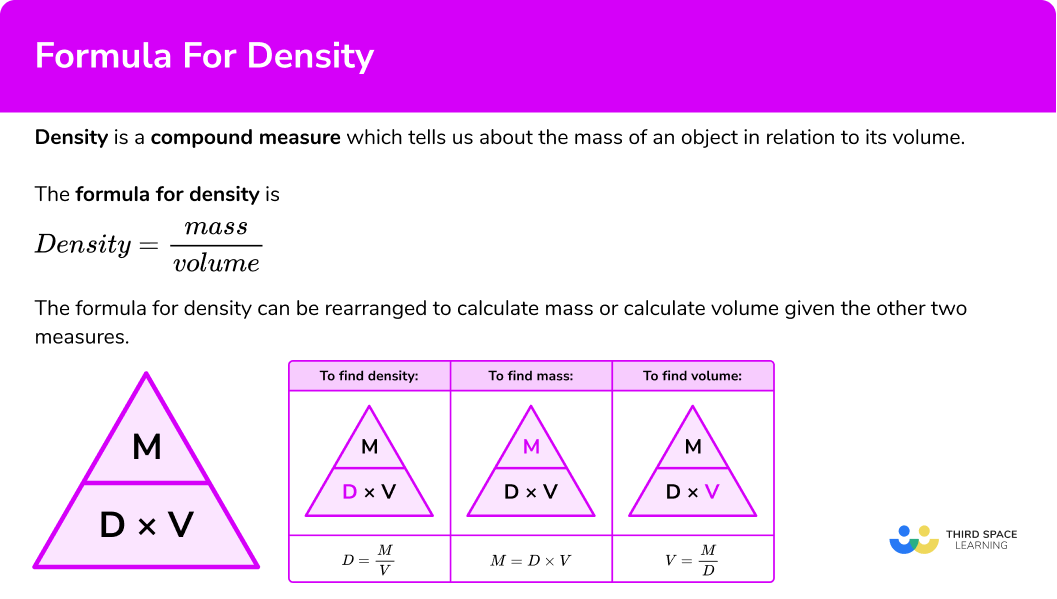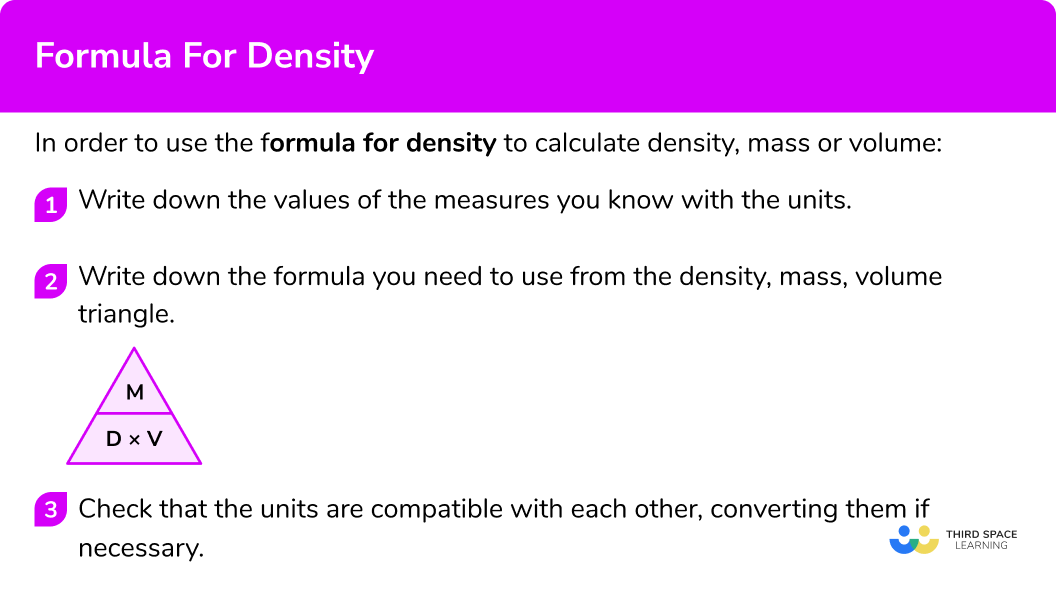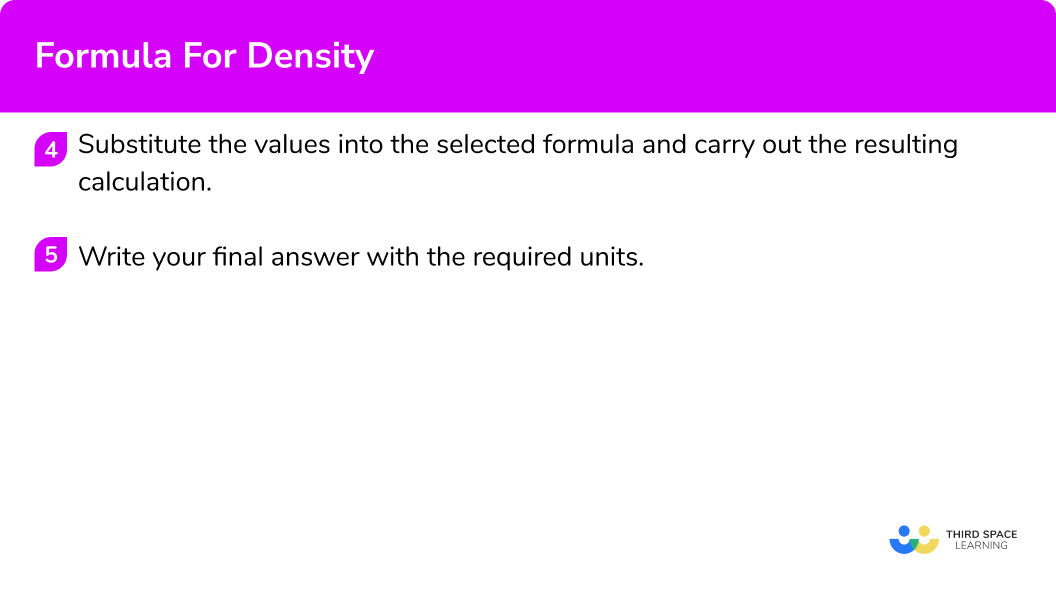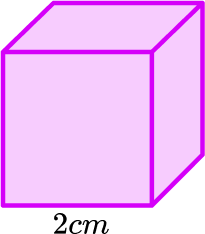# Formula For Density

Here we will learn about the formula for density, including how to calculate density, mass and volume and how to solve problems involving these measures.

There are also formula for density worksheets based on Edexcel, AQA and OCR exam questions, along with further guidance on where to go next if you’re still stuck.

## What is the formula for density?

The formula for density is:

\text{Density}=\frac{\text{mass}}{\text{volume}}.

“Density equals mass divided by volume.”

Density is a compound measure which tells us about the mass of an object in relation to its volume.

For example, if an object has a mass of 500 \ kg and a volume of 2.5 cubic metres then the density would be

\text{Density } = \text{ mass } \div \text{ volume } = 500 \div 2.5 = 200 \ kg/m^{3}.

The density formula can be rearranged to calculate mass or calculate volume given the other two measures. An easy way to remember the formula and the different rearrangements is to use this density mass volume triangle.

From this triangle we can work out how to calculate each measure. We can “cover up” what we are trying to find and the formula triangle tells us what calculation to do.

For example, if an object that has a density of 750 \ kg/m^{3} and a mass of 225 \ kg then its volume would be

\text{Volume } = \text{ mass } \div \text{ density } = 225 \div 750 = 0.3 \ m^{3}.

For example, if an object that has a density of 6.8 \ g/cm^3 and a volume of 2.7 \ g/cm^3, then its mass would be

\text{Mass } = \text{ density } \times \text{ volume } = 6.8 \times 2.7 = 18.36 \ grams.

### What is the formula for density?### Density, mass, volume and units of measure

Density is a compound measure and therefore involves two units; a combination of a mass in relation to volume.

It is very important to be aware of the units being used when using the density formula to calculate density, mass or volume.

• Examples of units of mass: grams (g), kilograms (kg), milligrams (mg).
• Examples of units of volume: cubic centimetres (cm^{3}), cubic metres (m^{3}), litres (l), millilitres (ml).
• Examples of units of density: grams per cubic centimetre g/cm^{3}, kilograms per cubic metre kg/m^{3}.

When you use the formula for density you must check that each measure is in the appropriate unit before you carry out the calculation. Sometimes you will need to convert a measure into different units. Here are some useful conversions to remember;

Units of Mass:

1 \ g = 1000 \ mg

1 \ kg = 1000 \ g

Units of Volume:

1\ m^{3}=1000000\ cm^{3}

1 \ litre = 1000 \ ml

1 \ ml=1 \ cm^{3}

1 \ litre = 1000 \ cm^{3}

For example, let’s calculate the density of an object that has a mass of 5300 \ g and a volume of 0.02 cubic metres.

For density the units that should be used are kg/m^{3} or g/cm^{3}.

The units given in this question are g and m^{3} so we need to convert one of them.

1000 \ g = 1 \ kg

Therefore,

5300 \ g = 5.3 \ kg.

Then we can calculate the density in kg/m^{3},

\text{Density } = \text{ mass } \div \text{ volume } = 5.3 \div 0.02 = 265\ kg/m^{3}.

Note also that sometimes you may need to convert an answer into different units at the end of a calculation.

### Density in science

Using the formula for density we can calculate the density of a variety of things such as the density of a material, the density of liquid, the density of air. The greek letter \rho (pronounced ‘rho’) is sometimes used to represent density.

In science there is an international system of units, known by the abbreviation SI.

This is the most modern form of the metric system and is most commonly used across the world.

The SI unit for mass is kilograms (kg).

The SI unit for length is metres (m).

The SI unit for volume is cubic metres (m^{3}).

These units would then calculate a density in kilograms per cubic metre (kg/m^{3}). However the density unit of grams per cubic centimetres (g/cm^{3}) is often used.

## How to use the formula for density

In order to use the formula for density to calculate density, mass or volume:

1. Write down the values of the measures you know with the units.
2. Write down the formula you need to use from the density, mass, volume triangle.
3. Check that the units are compatible with each other, converting them if necessary.
4. Substitute the values into the selected formula and carry out the resulting calculation.

### Explain how to use the formula for density### Explain how to use the formula for density### Related lessons oncompound measures

Formula for density is part of our series of lessons to support revision on compound measures. You may find it helpful to start with the main compound measures lesson for a summary of what to expect, or use the step by step guides below for further detail on individual topics. Other lessons in this series include:

## Formula for density examples

### Example 1: calculating density

A bar is made from aluminium.

It has volume 50\ cm^{3} and mass 135 \ g.

Calculate the density of the object.

1. Write down the values of the measures you know with the units.

\text{Density } = \ ?

\text{Mass } = 135 \ g

\text{Volume } = 50 \ cm^{3}

2Write down the formula you need to use from the density, mass, volume triangle.

We want to calculate density.

\text{Density}=\frac{\text{mass}}{\text{volume}}

3Check that the units are compatible with each other, converting them if necessary.

The units are g and cm^{3} which are compatible to calculate a density in g/cm^{3}.

4Substitute the values into the selected formula and carry out the resulting calculation.

\text{Density}=\frac{\text{mass}}{\text{volume}}=\frac{135}{50}=2.7

2.7 \ g/cm^{3}

### Example 2: calculating density

A block of ice has a volume of 576 \ cm^{3} and a mass of 530 \ g.

Calculate the density of the ice.

Write down the values of the measures you know with the units.

Write down the formula you need to use from the density, mass, volume triangle.

Check that the units are compatible with each other, converting them if necessary.

Substitute the values into the selected formula and carry out the resulting calculation.

### Example 3: calculating volume

A student weighs a piece of granite and records its mass as 800 \ g. The teacher tells the student that the density of the granite is 2.5\ g/cm^{3}.

Using this information, calculate the volume of the piece of granite.

Write down the values of the measures you know with the units.

Write down the formula you need to use from the density, mass, volume triangle.

Check that the units are compatible with each other, converting them if necessary.

Substitute the values into the selected formula and carry out the resulting calculation.

### Example 4: calculating volume with unit conversion

The mass of an object is 2.8 \ kg. The object is made of a substance which has a density of 5.2 \ g/cm^{3} .

Calculate the volume of the object.

Write down the values of the measures you know with the units.

Write down the formula you need to use from the density, mass, volume triangle.

Check that the units are compatible with each other, converting them if necessary.

Substitute the values into the selected formula and carry out the resulting calculation.

### Example 5: calculating mass with unit conversion

Liquid benzene has a density of 0.9 \ g/cm^{3}.

Find the mass of 5 \ litres of liquid benzene.

Write down the values of the measures you know with the units.

Write down the formula you need to use from the density, mass, volume triangle.

Check that the units are compatible with each other, converting them if necessary.

Substitute the values into the selected formula and carry out the resulting calculation.

### Example 6: calculating mass with unit conversion

Iron has a density of 7.8 \ g/cm^{3}.

Find the mass of 620 \ cm^{3} of iron.

Write down the values of the measures you know with the units.

Write down the formula you need to use from the density, mass, volume triangle.

Check that the units are compatible with each other, converting them if necessary.

Substitute the values into the selected formula and carry out the resulting calculation.

### Common misconceptions

• Thinking that density cannot be calculated because the volume is missing

When you are asked to calculate density you need to know what the mass and volume are. In some cases you will simply be given the volume but in other cases you may have to calculate the volume for yourself. Remember the volume of a cuboid is the product of its three dimensions.

For example,

\text{Volume}=3\times 4\times 8 = 96\ cm^3

Step-by-step guide: How to calculate volume

• Using incompatible units in a calculation

When using the formula for density you must ensure that the units of the measures are compatible. Density is usually measured in g/cm^{3} or kg/m^{3}. This table shows the compatible units.

So, for example, if you are given a mass in grams but a volume in m^{3} then these are not compatible and you must convert one of the measures.

Always think about the units of your final answer. Read the question carefully and check to see if any specific units are required. In some cases you may have to convert your answer into different units after a calculation.

Also check to see if the question requires your answer to be to a certain degree of accuracy such as one decimal place, or 2 significant figures.

### Practice formula for density questions

1. Find the density of an object with a mass of 900 \ g and a volume of 300 \ cm^{3}.

3 \ g/cm^3\frac{1}{3} \ g/cm^3270000 \ g/cm^30.3 \ kg/cm^3\text{Density}=\frac{\text{mass}}{\text{volume}}=\frac{900}{300}=3 \ g/cm^3

2. Find the density of an object which has a volume of 690 \ cm^3 and a mass of 950 \ g. Give your answer to 3 significant figures.

0.726 \ g/cm^30.727 \ g/cm^31.38 \ g/cm^31.37 \ g/cm^3\text{Density}=\frac{\text{mass}}{\text{volume}}=\frac{950}{690}=1.3768…=1.38 \ g/cm^3 (to 3 sf)

3. Find the volume of an object which has a mass of 600 \ g and a density of 12 \ g/cm^3.

0.02 \ cm^350 \ cm^37200 \ cm^30.02 \ m^3\text{Volume}=\frac{\text{mass}}{\text{density}}=\frac{600}{12}=50 \ cm^3

4. Find the volume of an object which has a density of 10.4 \ g/cm^3 and a mass of 230 \ g. Give your answer to 3 significant figures.

0.0452 \ cm^322.2 \ cm^322.1 \ cm^30.0453 \ cm^3\text{Volume}=\frac{\text{mass}}{\text{density}}=\frac{230}{10.4}=22.115…=22.1\ cm^3 (to 3 sf)

5. The density of a substance is 8 \ g/cm^3. If there is 480 \ cm^3 of this substance, what is the mass in kg?

0.60.63.843840\text{Mass}=\text{density}\times \text{volume}=8\times 480=3840 \ g

3840 \ g= 3.84 \ kg

6. The density of this cube is 11.2 \ g/cm^3. Find the mass.89.6 \ g22.4 \ g5.6 \ g1.4 \ gFirst we need the volume of the cube.

\text{Volume}=2\times 2\times 2=8\ cm^3

Then we can find the mass by multiplying the density by the volume.

\text{Mass}=\text{density}\times \text{volume}=11.2\times 8=89.6 \ g

### Formula for density GCSE questions

1. A bouncy ball is made from a special type of material.

The ball has a mass of 450 \ g.

The ball has a volume of 200 \ cm^3.

Calculate the density of the material.

(2 marks)

D=\frac{M}{V}=\frac{450}{200}=

(1)

2.25 \ g/cm^3

(1)

2. An iron bar has a mass of 25 \ kg.

The density of iron is 7.8 \ g/cm^3.

Work out the volume of the iron bar.

(3 marks)

25\times 1000=25 \ 000 \ g

(1)

V=\frac{M}{D}=\frac{25 \ 000}{7.8}=3205.1…

(1)

3210\ cm^3 (to 3 sf)

(1)

3. The density of orange juice is 1.3 \ g/cm^3.

The density of fizzy water is 0.98 \ g/cm^3.

30 \ cm^3 of orange juice is mixed with 220 \ cm^3 of fizzy water to make a fizzy orange drink.

Work out the density of the fizzy orange drink.

(4 marks)

1.3\times 30=39

0.98\times 220=215.6

For mass of orange juice OR mass of fizzy water

(1)

39+215.6=254.6

(1)

Total volume 30 + 220 = 250

D=\frac{M}{V}=\frac{254.6}{250}=1.0184

(1)

1.02\ g/cm^3 (to 3 sf)

(1)

## Learning checklist

You have now learned how to:

• Calculate the density of a substance
• Calculate the volume of an object given its mass and density
• Calculate the mass of an object given its volume and density
• Change between related standard units

## Still stuck?

Prepare your KS4 students for maths GCSEs success with Third Space Learning. Weekly online one to one GCSE maths revision lessons delivered by expert maths tutors.

Find out more about our GCSE maths tuition programme.